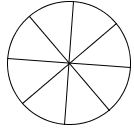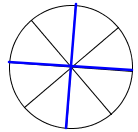### Home > CCA > Chapter A > Lesson A.1.6 > ProblemA-65

A-65.

Draw a circle on your paper and lightly shade in three-fourths of the circle.

1. Divide the entire circle into eight equal parts. How many parts are shaded?

• Try shading in three-fourths of the circle after you divide it into 8 equal parts. A circle divided into 8 equal sections cut through the center.

• Additional markers indicating the circle can be divided into quarters. Each quarter contains two sections of the 8 equal sections.1. Using fractions, write and solve a related division problem.

• Your problem should show how many one-eighth parts are in three-fourths.

• $\frac{3}{4}\div\frac{1}{8}$